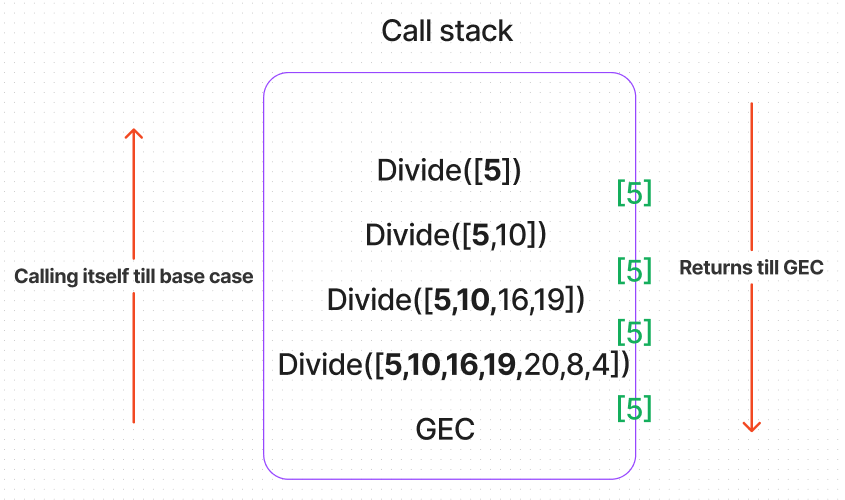top of page

# Algorithms using Recursion in Javascript

##### Divide and Conquer Algorithms:

Divide and Conquer is one of the algorithm paradigms that uses recursion.

Here,

• The large problem is divided into smaller problems - Divide.

• These smaller problems are of the same type.

• These smaller problems are solved recursively - Recursion.

• The results of smaller problems are combined to get the final solution - Conquer.

##### Dividing the array until it reaches the single value:

Take an array and divide it into half until it reaches a single value.

Getting a single value will be the base case.

Dividing the array will be a recursive case.

We will look into example,

```function Divide(arr) {
// evaluate the length of array
let arrLength = arr.length
// Base case
// Ifarray length is one
if(arrLength === 1 || arrLength === 1){
return arr
}

// Recursive case
// If array length greater than 1
// Divide the array
if(arrLength > 1) {

// Divide the array in to half
// Array length can be odd or even number
// If odd --> Math.ceil will give nearest greater integer
// e.g.Math.ceil(6.5) is 7

let midIndex = Math.ceil(arrLength / 2)

// We have two arrays
// Slice(start,end) --> start included and end excluded
// To include end --> index + 1 = arrLength

let leftArr = arr.slice(0,midIndex) // Takes only left half of array
return Divide(leftArr)
}
}

const array = [5,10,16,19,20,8,4]
Divide(array) // ```

The call stack would be,Similarly, for the right half of the array,

```function Divide(arr) {
// evaluate the length of array
let arrLength = arr.length
// Base case
// Ifarray length is one
if(arrLength === 1 || arrLength === 1){
return arr
}

// Recursive case
// If array length greater than 1
// Divide the array
if(arrLength > 1) {

// Divide the array in to half
// Array length can be odd or even number
// If odd --> Math.ceil will give nearest greater integer
// e.g.Math.ceil(6.5) is 7

let midIndex = Math.ceil(arrLength / 2)

// We have two arrays
// Slice(start,end) --> start included and end excluded
// To include end --> index + 1 = arrLength

// Takes only right half of array
let rightArr = arr.slice(midIndex,arrLength)
return Divide(rightArr)
}
}

const array = [5,10,16,19,20,8,4]
Divide(array) // ```

The call stack would be,If we observe, we actually reach left most elements and rightmost elements of an array, respectively.

If we need to get the value , just give index of 0 because at last we have array of length 1.
##### Binary Search:

We can search elements in an array using binary search.

Condition is array should be sorted.

So we need to give a sorted array.

Same divide approach until we hit an array of length 1.

For example, if we want to find 10 in [5,10,15,20,25,30,35,40,45,50].

We don't have to loop through all the elements in the array(Note: Array should be sorted)

Binary search is all about finding mid index value and searching only in array-half that contains that number.

Let me show you,

```function BinarySearch(arr,toFind,start,end) {

// If start index is greater than end , it is empty array
// Base case
if(start > end) {
return false
}

//Finding the mid index
let mid = Math.ceil((start + end)/2)

// Base case
// If Midinde value = toFInd value --> Return true
if(arr[mid] === toFind) return true

// Recursive case
if(arr[mid] > toFind) {
// The element should be in left array
// We give mid-1 because we already checked mid before
return BinarySearch(arr,toFind,start,mid - 1)
}
else {
// The element should be in right array
// We give mid+1 because we already checked mid before
return BinarySearch(arr,toFind,mid + 1,end)
}
}

const arr = [5,10,15,20,25,30,35,40,45,50]
console.log(BinarySearch(arr,10,0,arr.length -1)) // true```

The call stack would be,If we want to find 55, then the call stack would be,See All
bottom of page